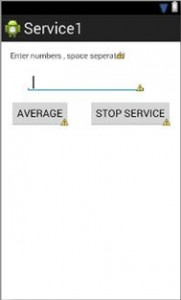# Implementing Simple Service in Android

0
2142In this tutorial we are going to implement a Simple Service which we have to start and stop.

• In this implementation of Service which will calculate the average of the series of number’s that we will enter in the text field.
• Steps are as Follows:
1. Create a new project , your MainActivity.java and activity_main.xml files will be created.
2. In the activity_main.xml file take a Large Text from the Palette.
3. Also take the Plain Text from the Text Fields and two buttons in the activity_Main.xml file.
4. Set the button property as : android:text=”Stop Service”.
5. And for another button set as : android:text=”Average”.
6. So now the text of the buttons are set as Stop Service and Average .
7. Now set the text in the text property of the TextView.
8. For example : android:text=”Enter Numbers ,Space Seperated”.
9. So Now your activity_main file’s Graphical Layout will look like this :
10.11. Now go to your MainActivity.java file .
12. In this declare the following in the MainACtivity class as :
1. Private EditText numbersText ;
2. Private static Intent averagingIntent ;

13. Now in the onCreate( ) method insert the following code :
numbersText = (EditText) findViewById(R.id.editNumbers);
14. So the MainActivity.java file will look for the EditText by finding it by its id.
15. So the MainActivity.java file will look like this :
16. Now write the following code for Average button in the MainActivity.java file as shown below :
17. [code language=”java”]public void startService(View v)
{
String[] strNumbers = numbersText .getText().toString().split(" ");
int[] numbers = new int[strNumbers.length];
for(int i=0;i<=strNumbers.length;i++)
{
numbers[i]=Integer.parseInt(strNumbers[i]);
}

averagingIntent = new Intent(MainActivity.this,TestService.class);
averagingIntent.putExtra("numbers", numbers);
startService(averagingIntent);
}

[/code]

18. Now startService is the name given to the onClick property of the Average button.
19. Let’s see the explanation of the code line by line :
1. startService gets a String array of numbers from the numbersText,getting its text ( ) ,converting it to string( ) and then splitting it on the space character.
2. Here we take the string array and convert it to the integer array by doing new int and the [strNumbers.length].
3. We take a for loop to set the integer array to the numbers entered by the user .
4. Now set up the intent by using the calling class and the target class and startService with the intent.

20. Then stop the service.
21. So now you will have the following code :
22. Now take another java class for example: TestService.java
23. Class TestService must be extended with Service .
24. In this write the oncreate( ) method which must be called through super.
25. [code language=”java”]public void onCreate()
{
super.onCreate();
}
[/code]

26. The next method important here is the onStartCommand this is where the intent enters into the service.
27. [code language=”java”]public int onstartCommand(Intent intent,int flags, int startId)
{
int[] numArray = intent.getExtras().getIntArray("numbers");
float avg = findAverage(numArray);
Toast.makeText(getApplicationContext(), String.format("Average : %f", avg), Toast.LENGTH_SHORT).show();

return startId;

}
[/code]

28. Here we get the numeric array an integer array by getting those extras with that number array.
29. Then the avg is the calculated average that is calculated by the “ find average logic” .
30. Toast is used to display the message of the calculated average.
31. Then return the start Id.
32. Also call the onDestroy( ) method to stop the service .
33. Write down the logic for the calculation of average.
34. [code language=”java”]float findAverage(int[]nums)
{
int sum=0;
for(int i=0;i<=nums.length;i++)
{
sum += nums[i];
}
return(float)sum/nums.length;
}

[/code]

35. So your window will look as shown below :
36. Now in the android manifest file list the service as stated
37. [code language=”xml”]<service
android:name=".TestService">
</service>
[/code]